# NCERT Exemplar Class 11 Chemistry Solutions for Chapter 12 - Organic Chemistry Solutions Some Basic Principles and Techniques

## NCERT Exemplar Solutions Class 11 Chemistry Chapter 12 – Free PDF Download

NCERT Exemplar Chemistry Class 11 Chapter 12 Organic Chemistry: Some Basic Principles and Techniques will help you clear the concepts involved in this chapter. In order to clear all your doubts on this chapter, students are advised to get thorough with concepts of NCERT Exemplar Chemistry Class 11 Chapter 12. NCERT Exemplar Class 11 Chemistry Chapter 12 PDF provides answers to the questions in NCERT Exemplar book together with important questions from previous year papers and sample papers, important structural formulas, exercises, worksheets, tips and tricks.

Organic Chemistry is one of the most important parts of chemistry. Organic chemistry is the chemistry sub-discipline for the scientific study of structure, properties, and reactions of organic compounds and organic materials. In Class 11, students will learn the basics of organic chemistry and the advanced concepts will be taught in Class 12.

### Download PDF of NCERT Exemplar Chemistry Class 11 Chapter 12 Organic Chemistry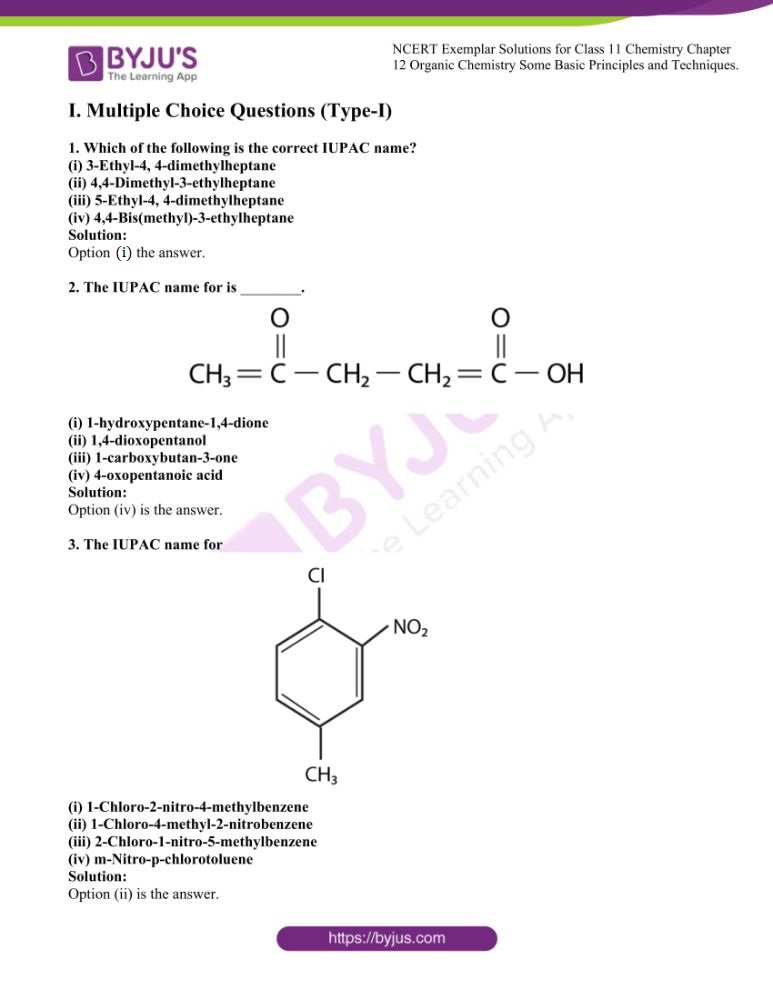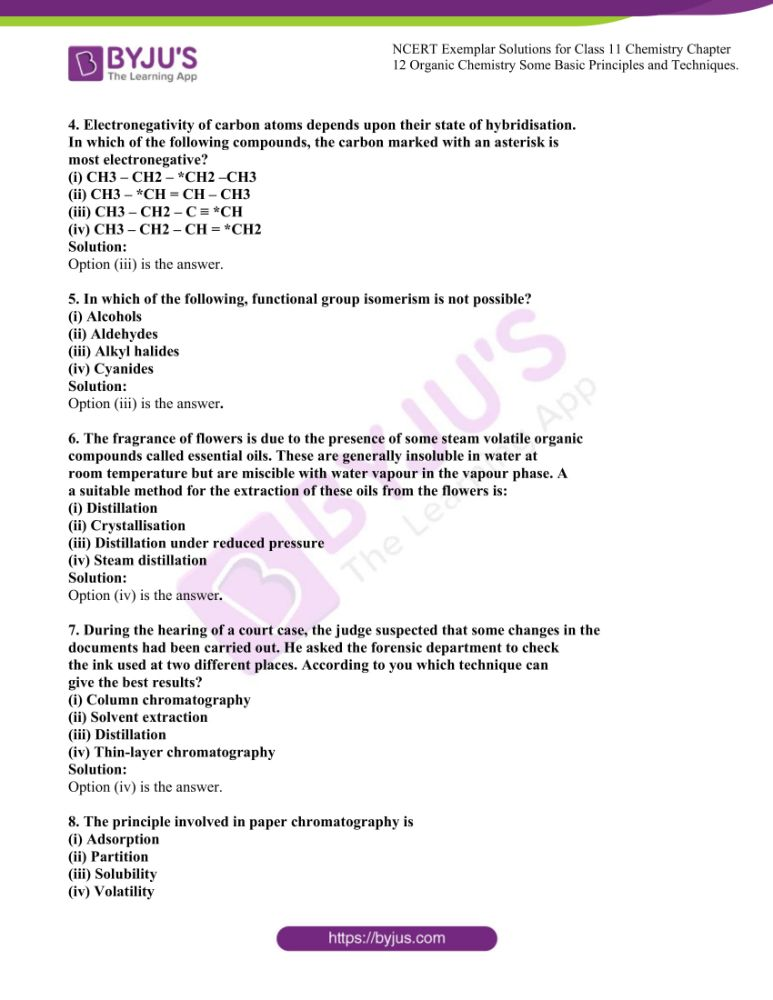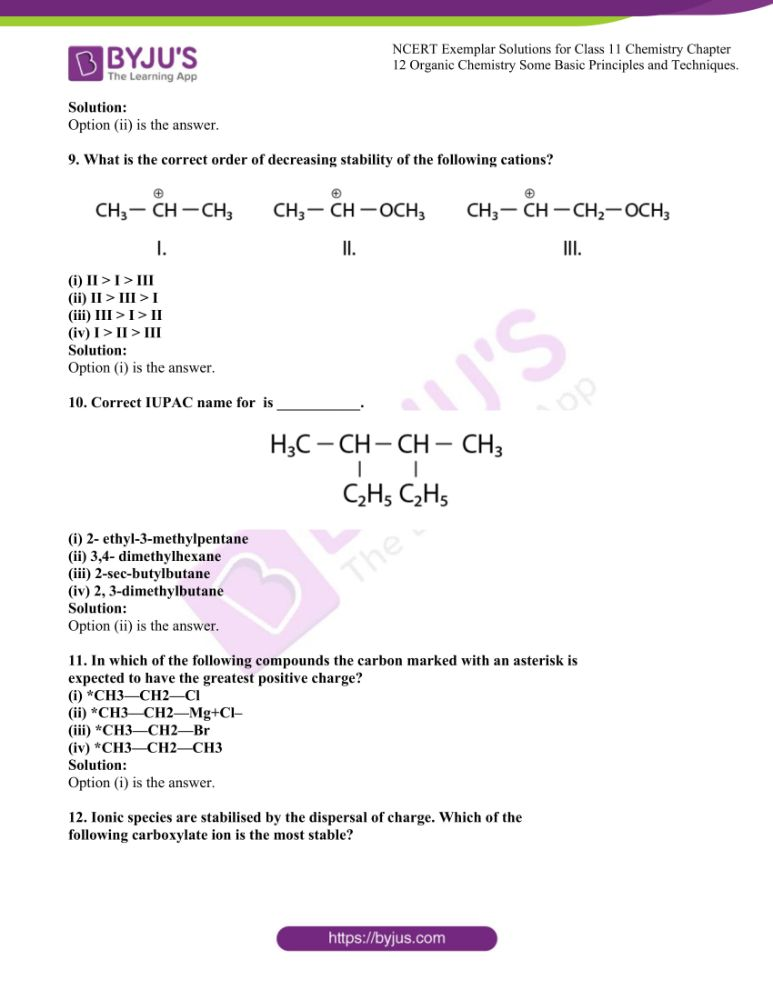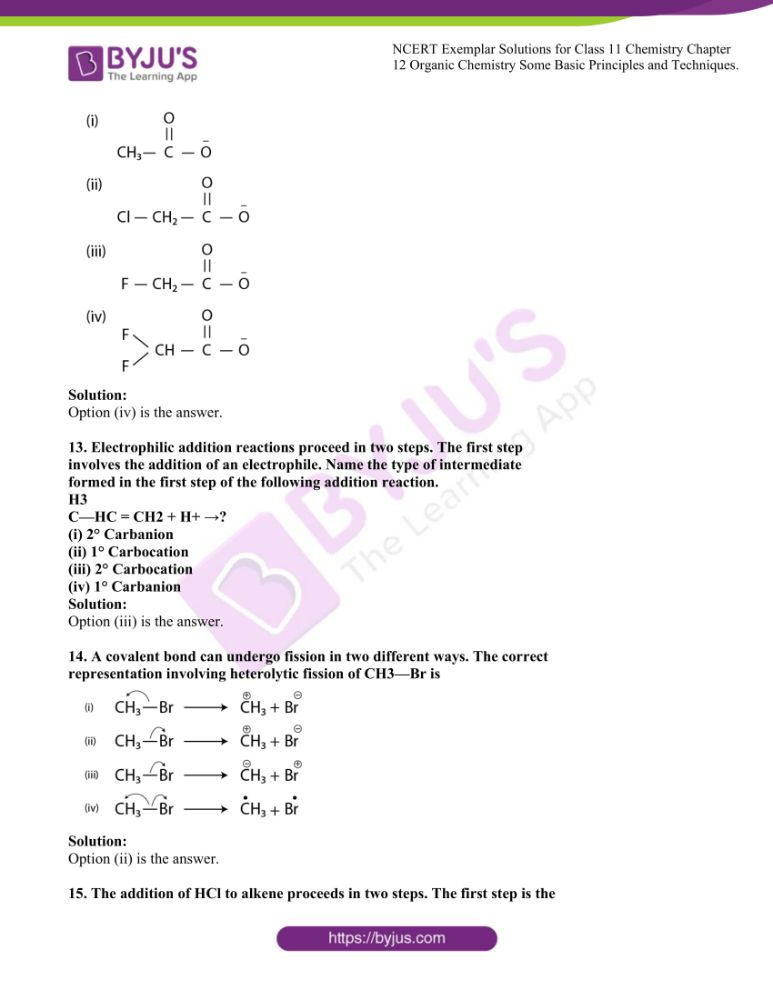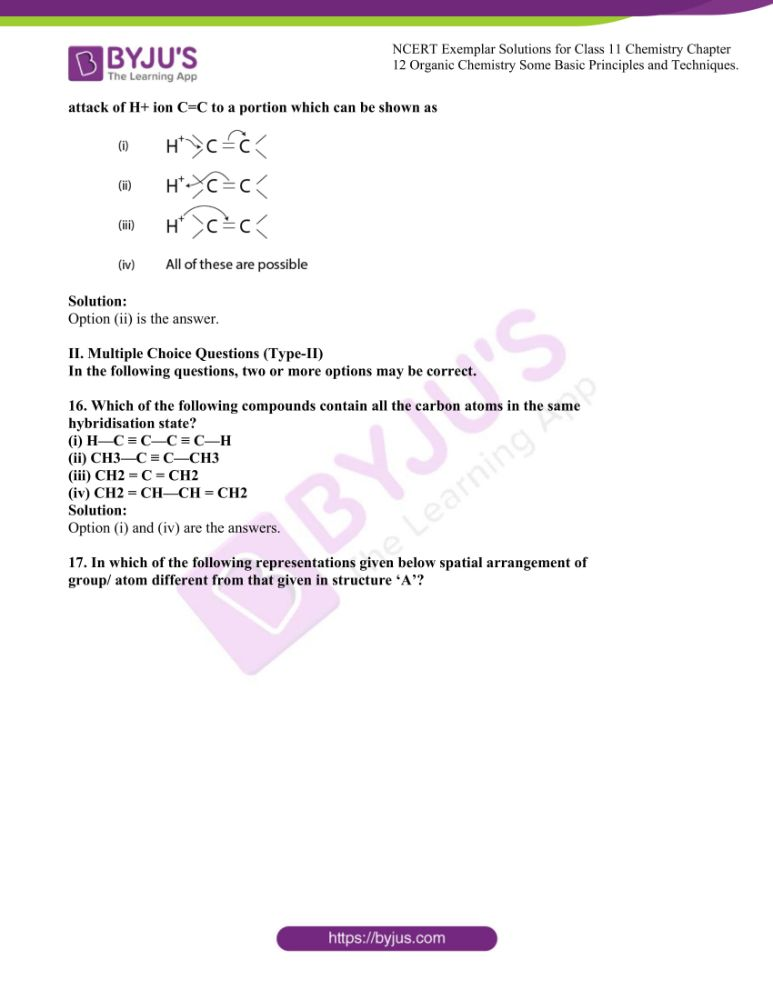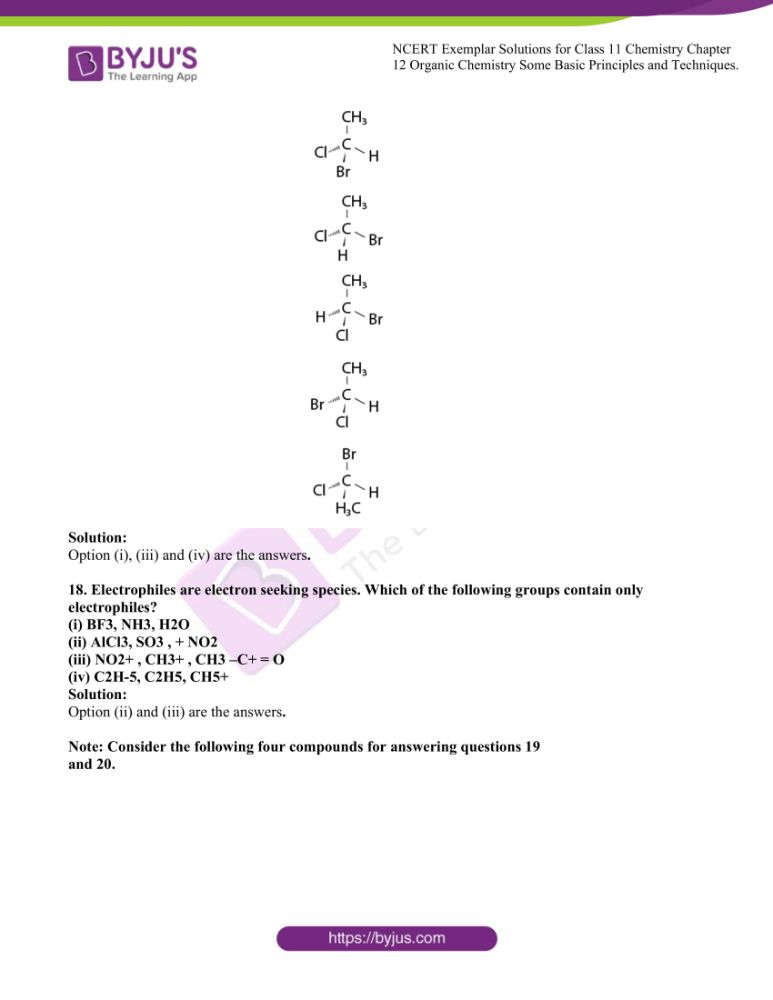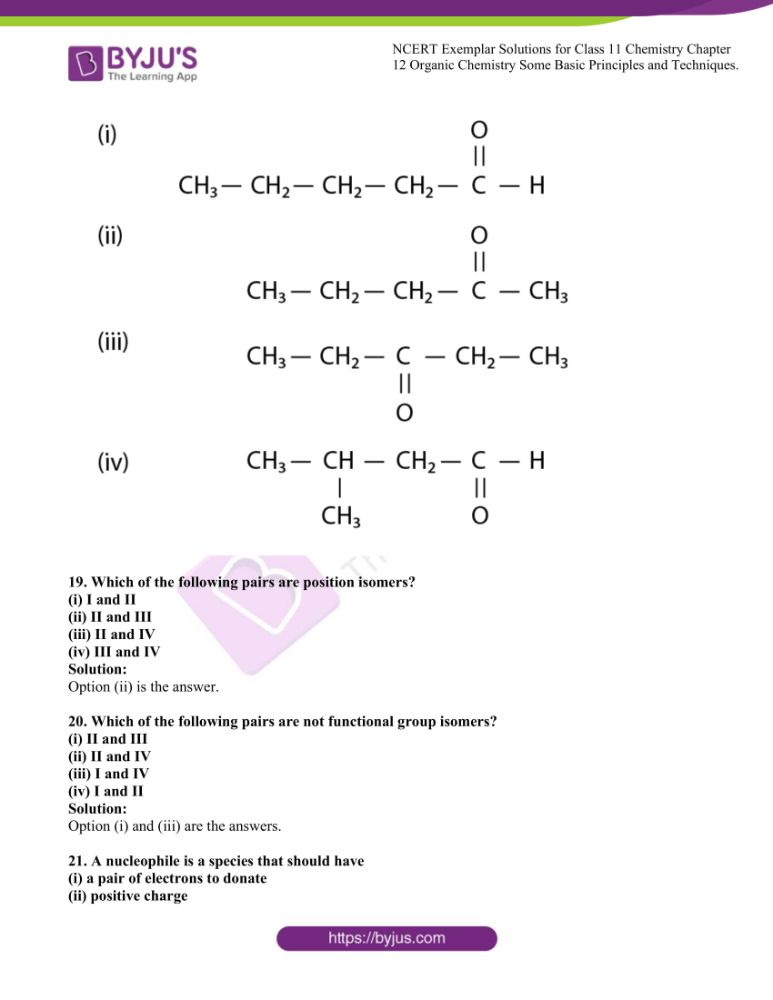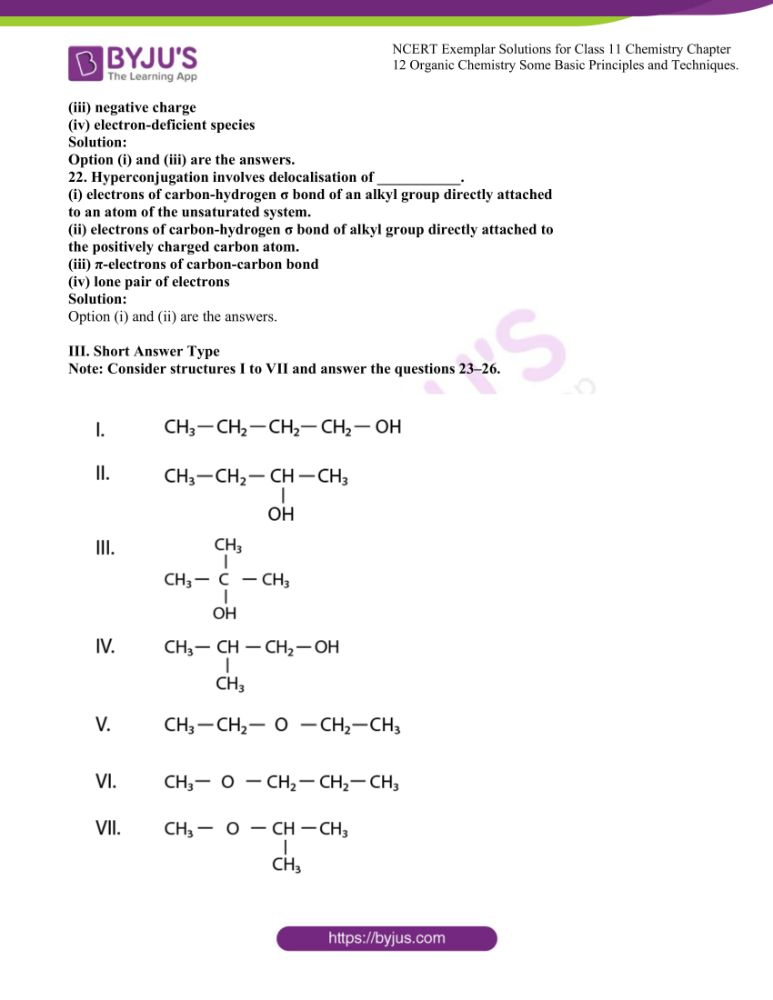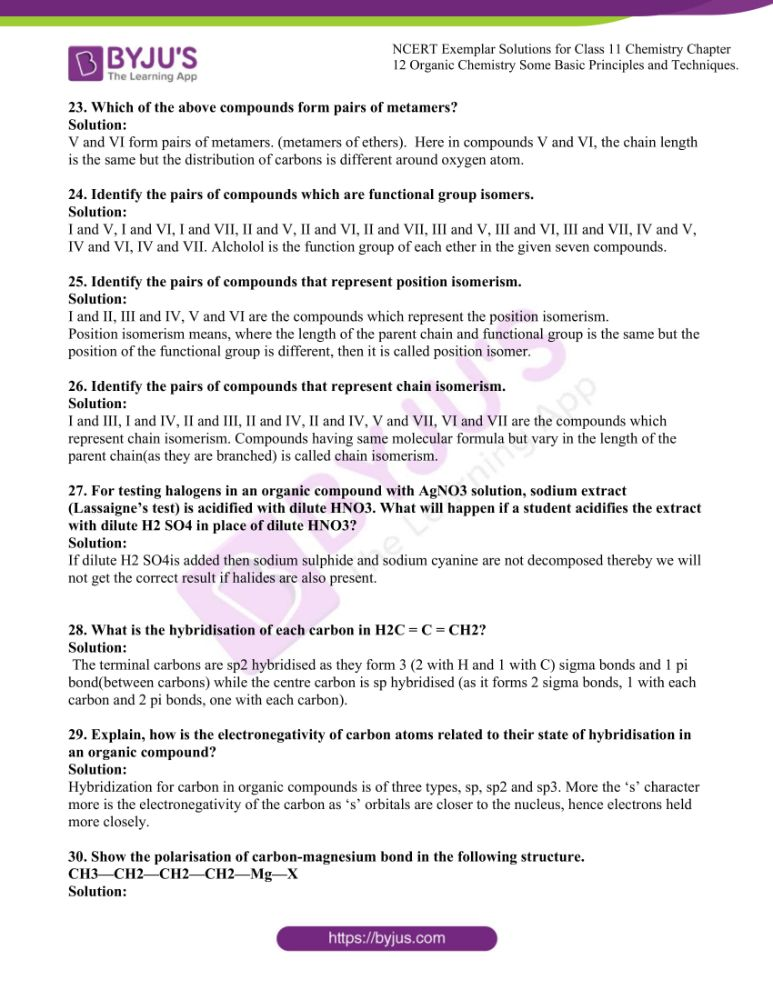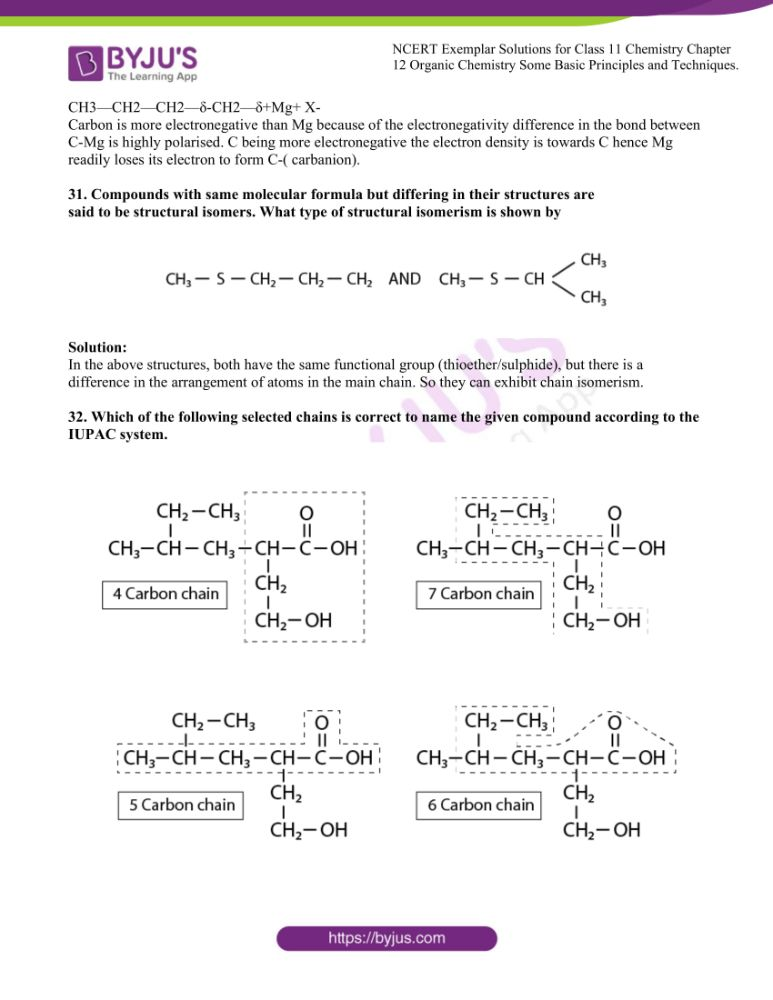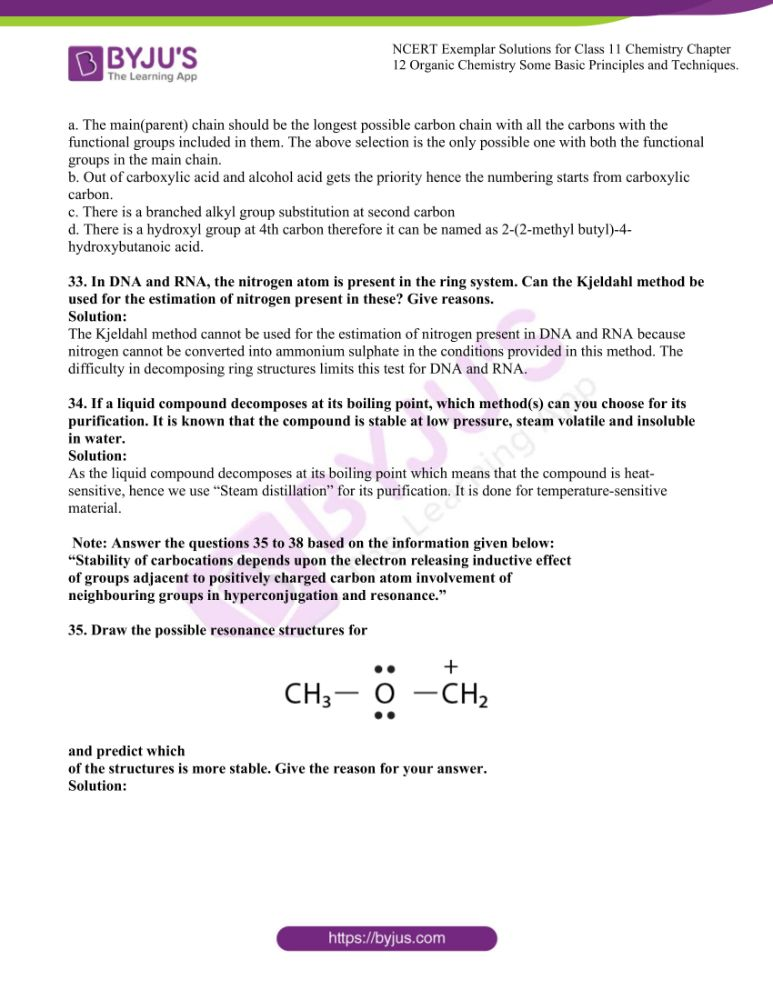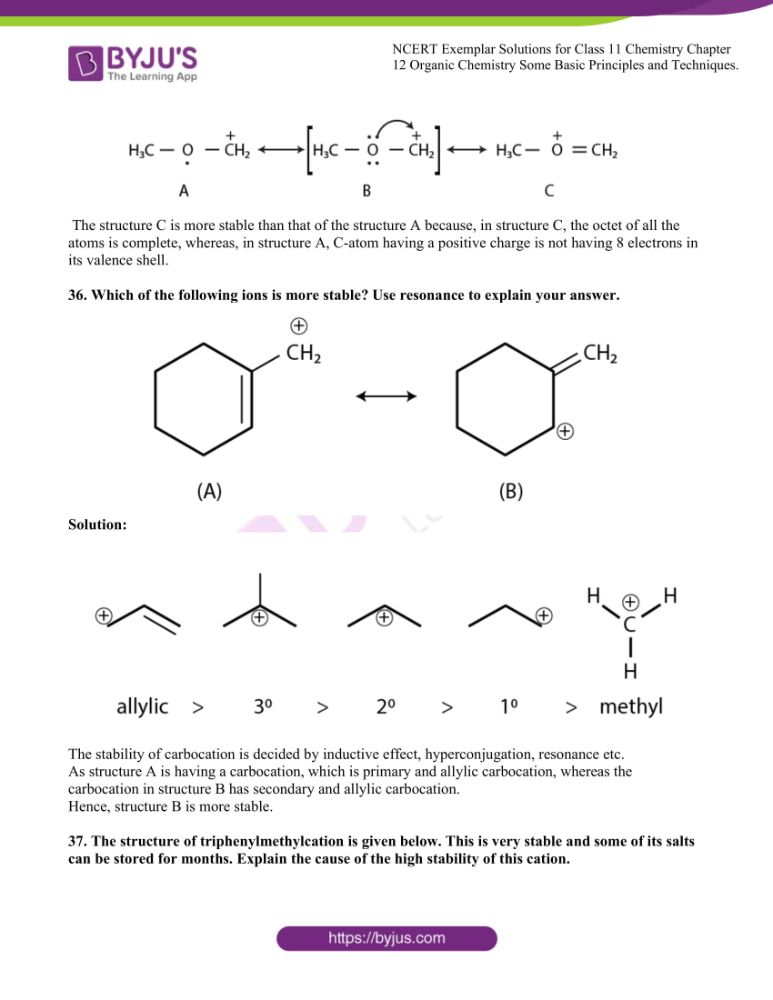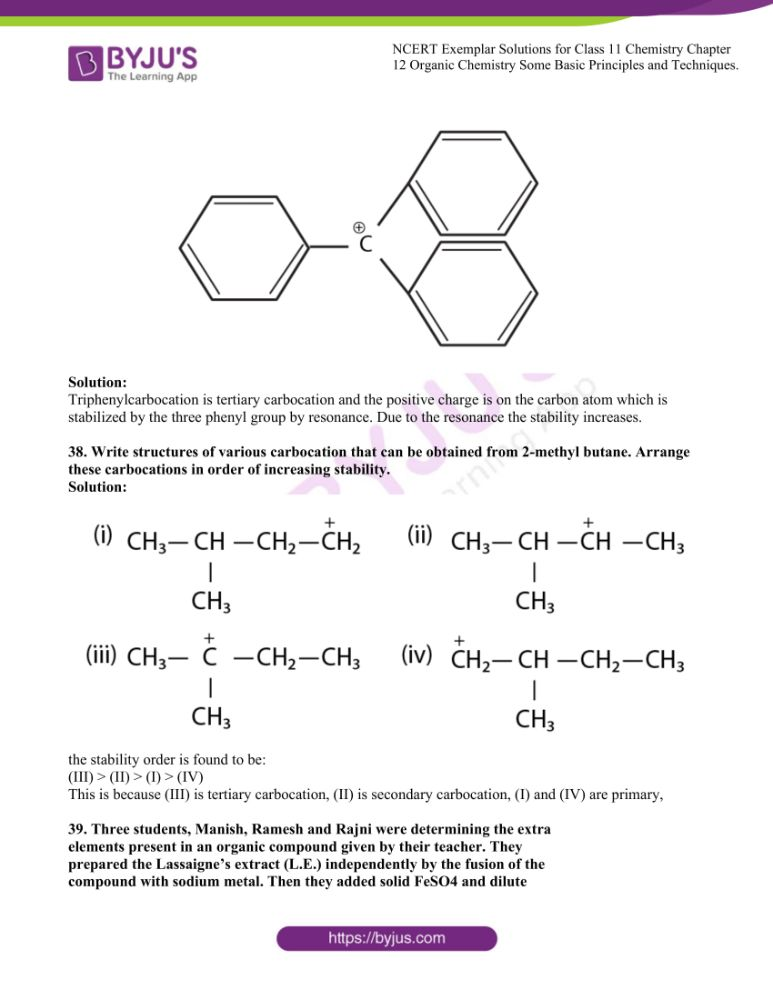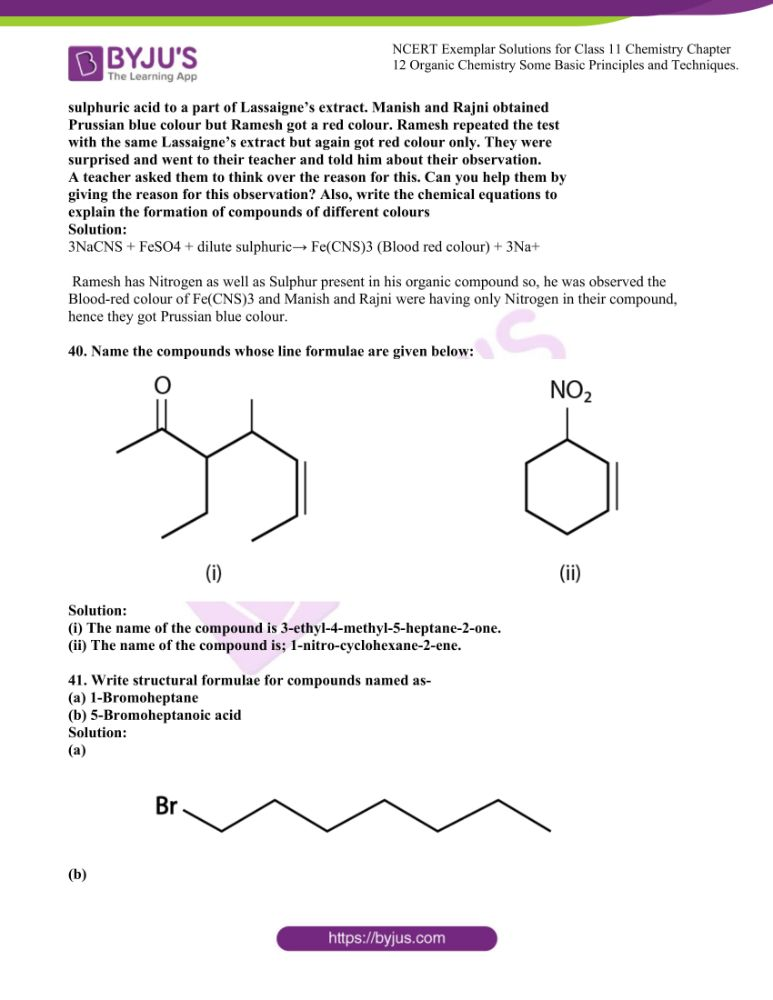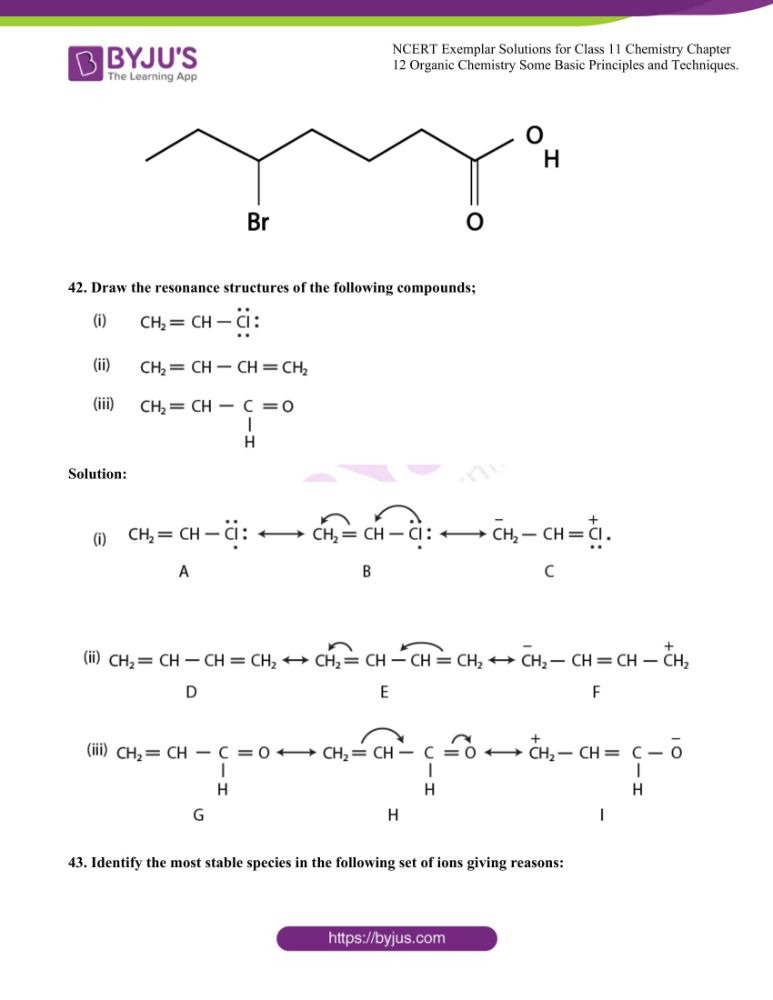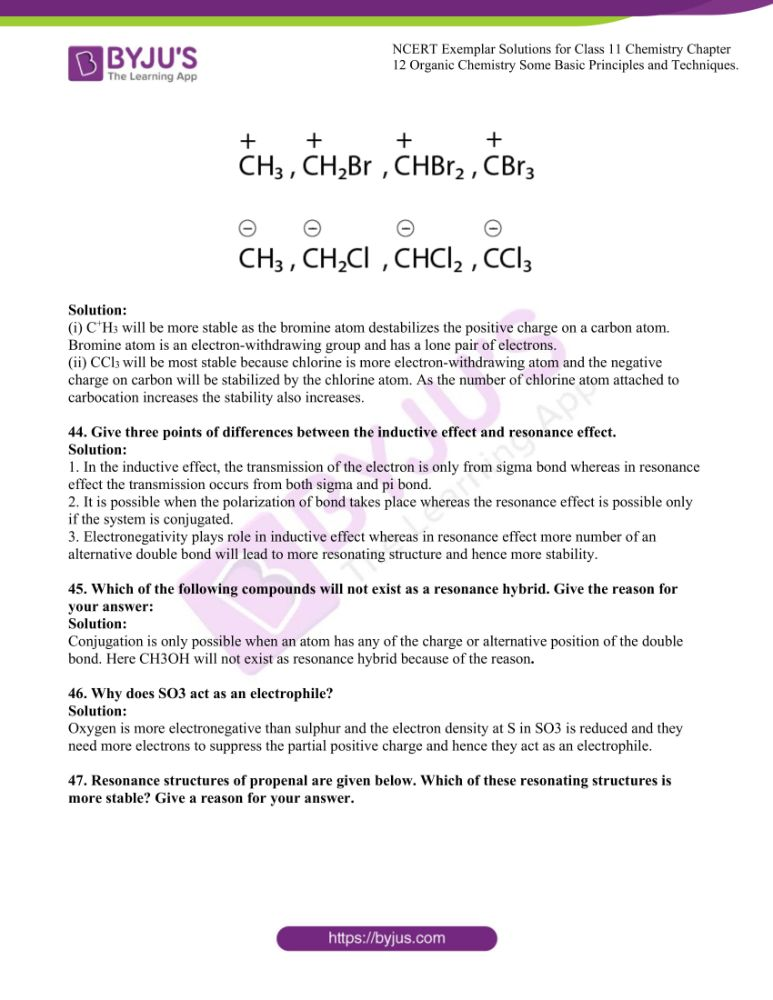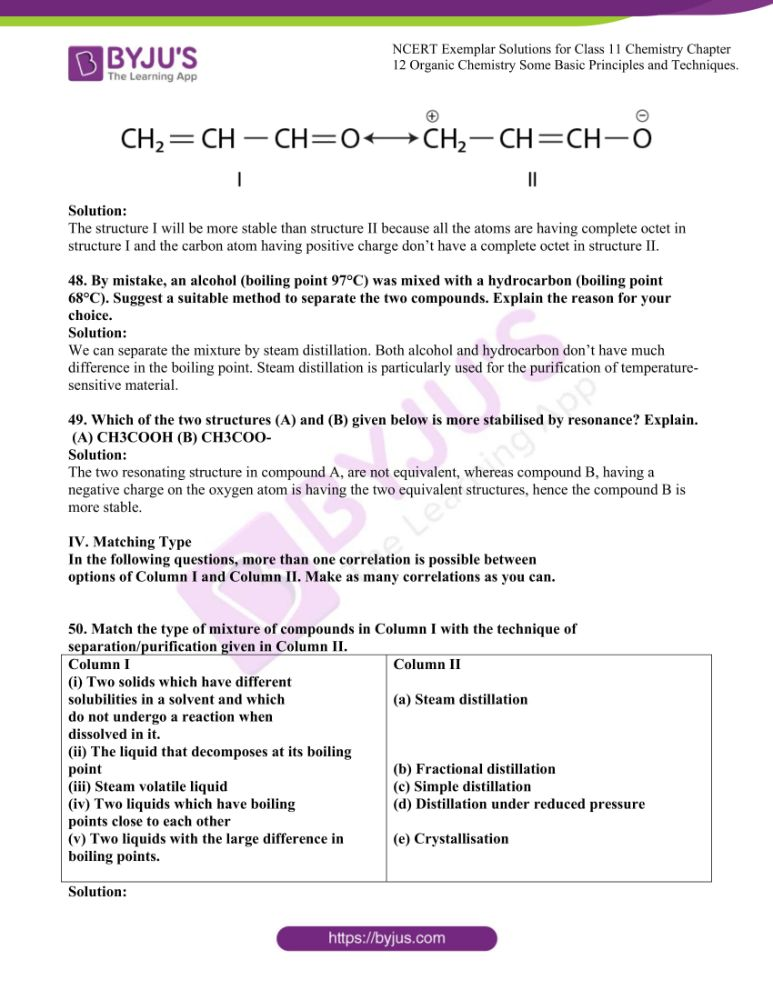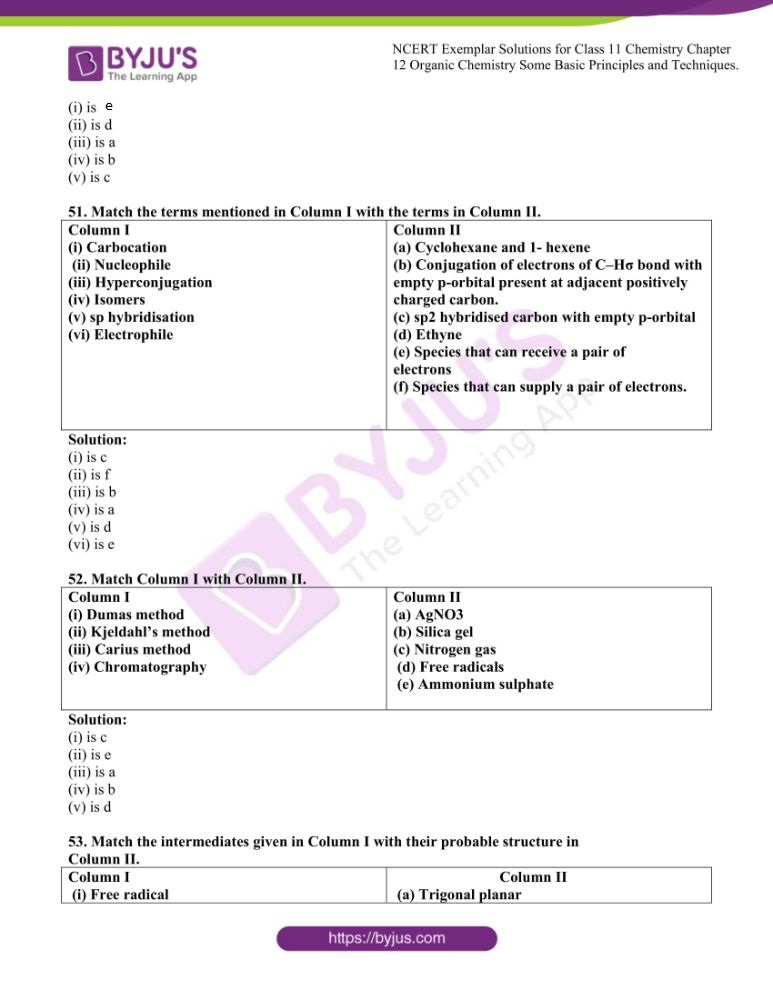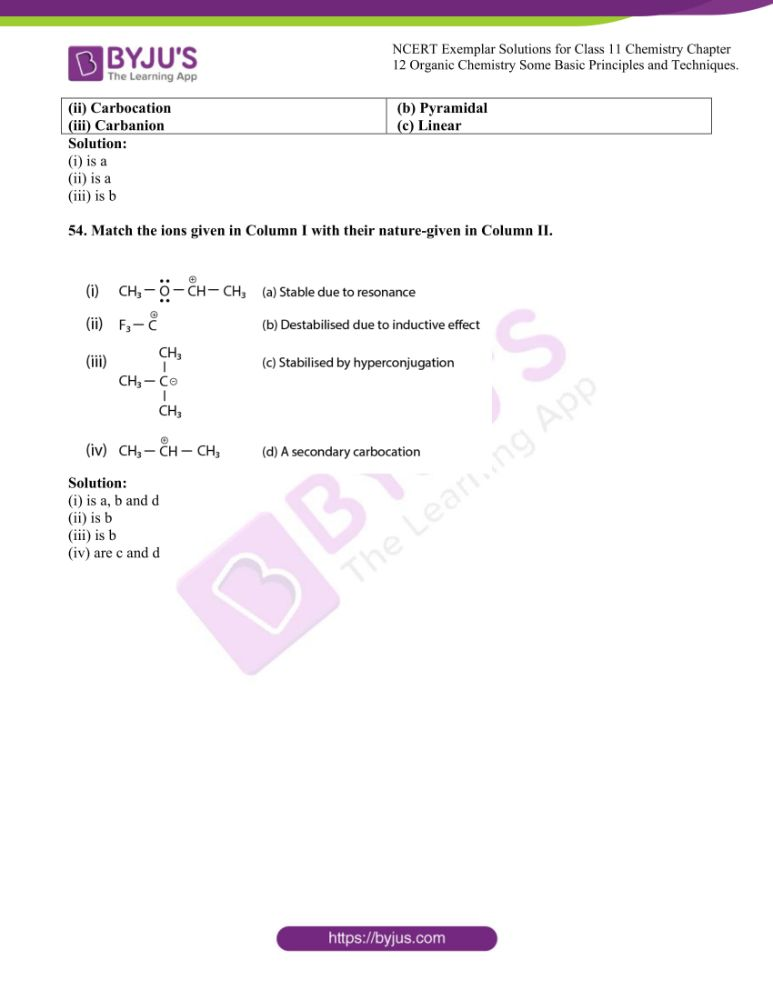### Access NCERT Exemplar Solutions for Chemistry Class 11 Chapter 12

I. Multiple Choice Questions (Type-I)

1. Which of the following is the correct IUPAC name?

(i) 3-Ethyl-4, 4-dimethylheptane

(ii) 4,4-Dimethyl-3-ethylheptane

(iii) 5-Ethyl-4, 4-dimethylheptane

(iv) 4,4-Bis(methyl)-3-ethylheptane

Solution:

Option (i) the answer.

2. The IUPAC name for is ________.(i) 1-hydroxypentane-1,4-dione

(ii) 1,4-dioxopentanol

(iii) 1-carboxybutan-3-one

(iv) 4-oxopentanoic acidSolution:

Option (iv) is the answer.

3. The IUPAC name for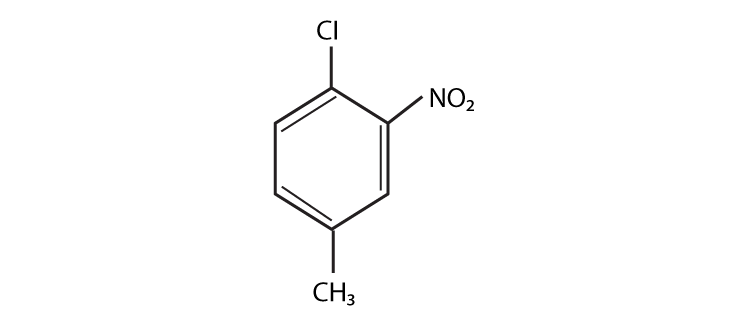(i) 1-Chloro-2-nitro-4-methylbenzene

(ii) 1-Chloro-4-methyl-2-nitrobenzene

(iii) 2-Chloro-1-nitro-5-methylbenzene

(iv) m-Nitro-p-chlorotoluene

Solution:

Option (ii) is the answer.

4. Electronegativity of carbon atoms depends upon their state of hybridisation.

In which of the following compounds, the carbon marked with an asterisk is

most electronegative?

(i) CH3 – CH2 – *CH2 –CH3

(ii) CH3 – *CH = CH – CH3

(iii) CH3 – CH2 – C ≡ *CH

(iv) CH3 – CH2 – CH = *CH2

Solution:

Option (iii) is the answer.

5. In which of the following, functional group isomerism is not possible?

(i) Alcohols

(ii) Aldehydes

(iii) Alkyl halides

(iv) Cyanides

Solution:

Option (iii) is the answer.

6. The fragrance of flowers is due to the presence of some steam volatile organic

compounds called essential oils. These are generally insoluble in water at

room temperature but are miscible with water vapour in the vapour phase. A

a suitable method for the extraction of these oils from the flowers is:

(i) Distillation

(ii) Crystallisation

(iii) Distillation under reduced pressure

(iv) Steam distillation

Solution:

Option (iv) is the answer.

7. During the hearing of a court case, the judge suspected that some changes in the

documents had been carried out. He asked the forensic department to check

the ink used at two different places. According to you which technique can

give the best results?

(i) Column chromatography

(ii) Solvent extraction

(iii) Distillation

(iv) Thin-layer chromatography

Solution:

Option (iv) is the answer.

8. The principle involved in paper chromatography is

(ii) Partition

(iii) Solubility

(iv) Volatility

Solution:

Option (ii) is the answer.

9. What is the correct order of decreasing stability of the following cations?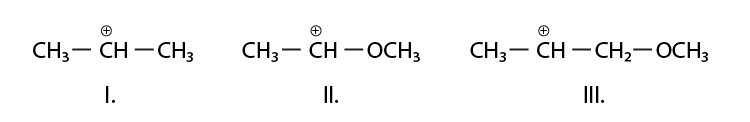(i) II > I > III

(ii) II > III > I

(iii) III > I > II

(iv) I > II > III

Solution:

Option (i) is the answer.

10. Correct IUPAC name for is ___________.(i) 2- ethyl-3-methylpentane

(ii) 3,4- dimethylhexane

(iii) 2-sec-butylbutane

(iv) 2, 3-dimethylbutane

Solution:

Option (ii) is the answer.

11. In which of the following compounds the carbon marked with an asterisk is

expected to have the greatest positive charge?

(i) *CH3—CH2—Cl

(ii) *CH3—CH2—Mg+Cl–

(iii) *CH3—CH2—Br

(iv) *CH3—CH2—CH3

Solution:

Option (i) is the answer.

12. Ionic species are stabilised by the dispersal of charge. Which of the

following carboxylate ion is the most stable?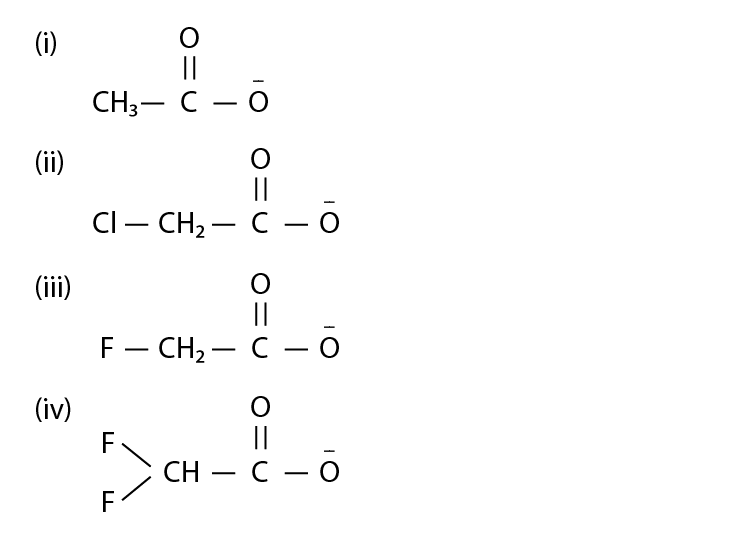Solution:

Option (iv) is the answer.

13. Electrophilic addition reactions proceed in two steps. The first step

involves the addition of an electrophile. Name the type of intermediate

formed in the first step of the following addition reaction.

H3

C—HC = CH2 + H+ →?

(i) 2° Carbanion

(ii) 1° Carbocation

(iii) 2° Carbocation

(iv) 1° Carbanion

Solution:

Option (iii) is the answer.

14. A covalent bond can undergo fission in two different ways. The correct

representation involving heterolytic fission of CH3—Br is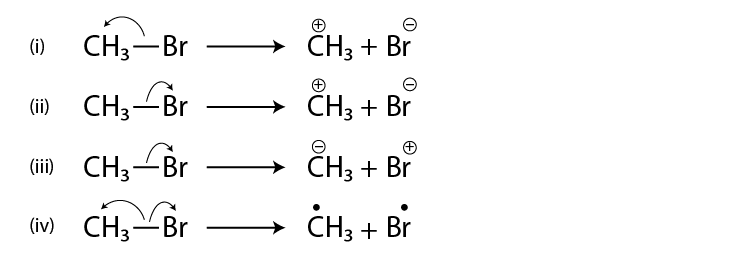Solution:

Option (ii) is the answer.

15. The addition of HCl to alkene proceeds in two steps. The first step is the

attack of H+ ion C=C to a portion which can be shown as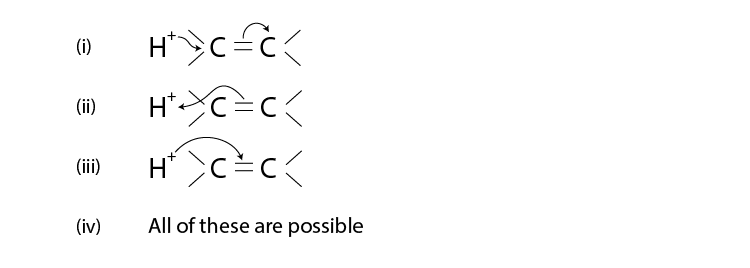Solution:

Option (ii) is the answer.

II. Multiple Choice Questions (Type-II)

In the following questions, two or more options may be correct.

16. Which of the following compounds contain all the carbon atoms in the same

hybridisation state?

(i) H—C ≡ C—C ≡ C—H

(ii) CH3—C ≡ C—CH3

(iii) CH2 = C = CH2

(iv) CH2 = CH—CH = CH2

Solution:

Option (i) and (iv) are the answers.

17. In which of the following representations given below spatial arrangement of

group/ atom different from that given in structure ‘A’?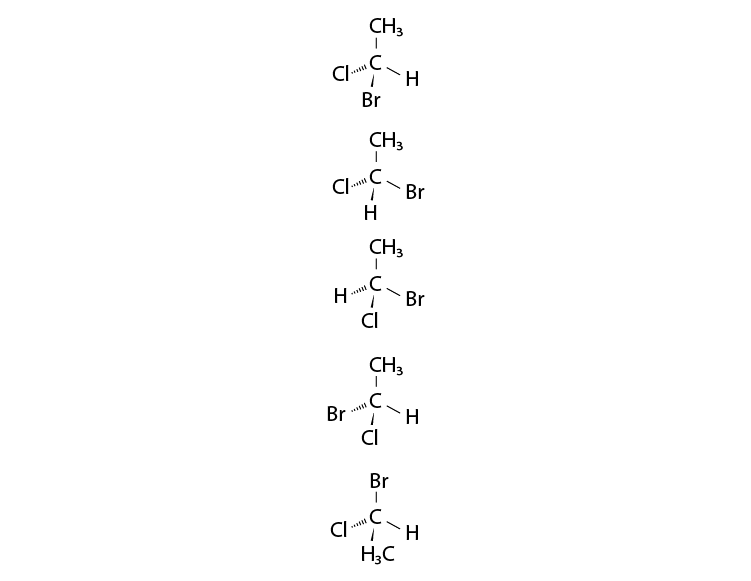Solution:

Option (i), (iii) and (iv) are the answers.

18. Electrophiles are electron seeking species. Which of the following groups contain only electrophiles?

(i) BF3, NH3, H2O

(ii) AlCl3, SO3 , + NO2

(iii) NO2+ , CH3+ , CH3 –C+ = O

(iv) C2H-5, C2H5, CH5+

Solution:

Option (ii) and (iii) are the answers.

Note: Consider the following four compounds for answering questions 19

and 20.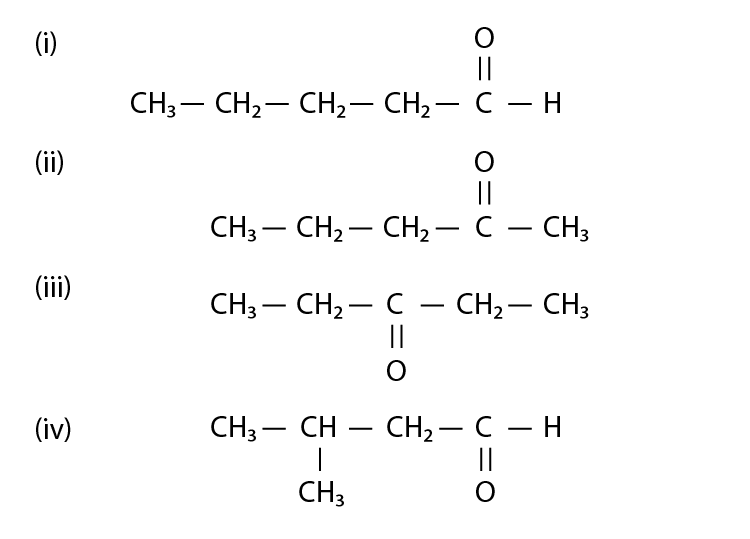19. Which of the following pairs are position isomers?

(i) I and II

(ii) II and III

(iii) II and IV

(iv) III and IV

Solution:

Option (ii) is the answer.

20. Which of the following pairs are not functional group isomers?

(i) II and III

(ii) II and IV

(iii) I and IV

(iv) I and II

Solution:

Option (i) and (iii) are the answers.

21. A nucleophile is a species that should have

(i) a pair of electrons to donate

(ii) positive charge

(iii) negative charge

(iv) electron-deficient speciesSolution:

Option (i) and (iii) are the answers.

22. Hyperconjugation involves delocalisation of ___________.

(i) electrons of carbon-hydrogen σ bond of an alkyl group directly attached

to an atom of the unsaturated system.

(ii) electrons of carbon-hydrogen σ bond of alkyl group directly attached to

the positively charged carbon atom.

(iii) π-electrons of carbon-carbon bond

(iv) lone pair of electrons

Solution:

Option (i) and (ii) are the answers.

III. Short Answer Type

Note: Consider structures I to VII and answer the questions 23–26.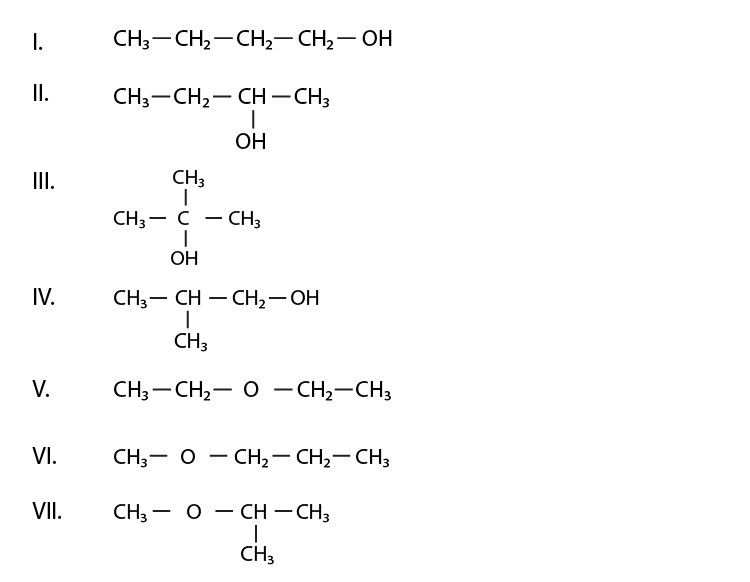23. Which of the above compounds form pairs of metamers?

Solution:

V and VI form pairs of metamers. (metamers of ethers). Here in compounds V and VI, the chain length is the same but the distribution of carbons is different around oxygen atom.

24. Identify the pairs of compounds which are functional group isomers.

Solution:

I and V, I and VI, I and VII, II and V, II and VI, II and VII, III and V, III and VI, III and VII, IV and V, IV and VI, IV and VII. Alcholol is the function group of each ether in the given seven compounds.

25. Identify the pairs of compounds that represent position isomerism.

Solution:

I and II, III and IV, V and VI are the compounds which represent the position isomerism.

Position isomerism means, where the length of the parent chain and functional group is the same but the position of the functional group is different, then it is called position isomer.

26. Identify the pairs of compounds that represent chain isomerism.

Solution:

I and III, I and IV, II and III, II and IV, II and IV, V and VII, VI and VII are the compounds which represent chain isomerism. Compounds having same molecular formula but vary in the length of the parent chain(as they are branched) is called chain isomerism.

27. For testing halogens in an organic compound with AgNO3 solution, sodium extract (Lassaigne’s test) is acidified with dilute HNO3. What will happen if a student acidifies the extract with dilute H2 SO4 in place of dilute HNO3?

Solution:

If dilute H2 SO4is added then sodium sulphide and sodium cyanine are not decomposed thereby we will not get the correct result if halides are also present.

28. What is the hybridisation of each carbon in H2C = C = CH2?

Solution:

The terminal carbons are sp2 hybridised as they form 3 (2 with H and 1 with C) sigma bonds and 1 pi bond(between carbons) while the centre carbon is sp hybridised (as it forms 2 sigma bonds, 1 with each carbon and 2 pi bonds, one with each carbon).

29. Explain, how is the electronegativity of carbon atoms related to their state of hybridisation in an organic compound?

Solution:

Hybridization for carbon in organic compounds is of three types, sp, sp2 and sp3. More the ‘s’ character more is the electronegativity of the carbon as ‘s’ orbitals are closer to the nucleus, hence electrons held more closely.

30. Show the polarisation of carbon-magnesium bond in the following structure.

CH3—CH2—CH2—CH2—Mg—X

Solution:

CH3—CH2—CH2—δ-CH2—δ+Mg+ X-

Carbon is more electronegative than Mg because of the electronegativity difference in the bond between C-Mg is highly polarised. C being more electronegative the electron density is towards C hence Mg readily loses its electron to form C-( carbanion).

31. Compounds with same molecular formula but differing in their structures are

said to be structural isomers. What type of structural isomerism is shown by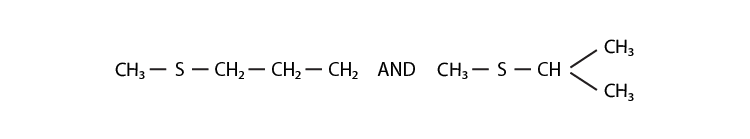Solution:

In the above structures, both have the same functional group (thioether/sulphide), but there is a difference in the arrangement of atoms in the main chain. So they can exhibit chain isomerism.

32. Which of the following selected chains is correct to name the given compound according to the IUPAC system.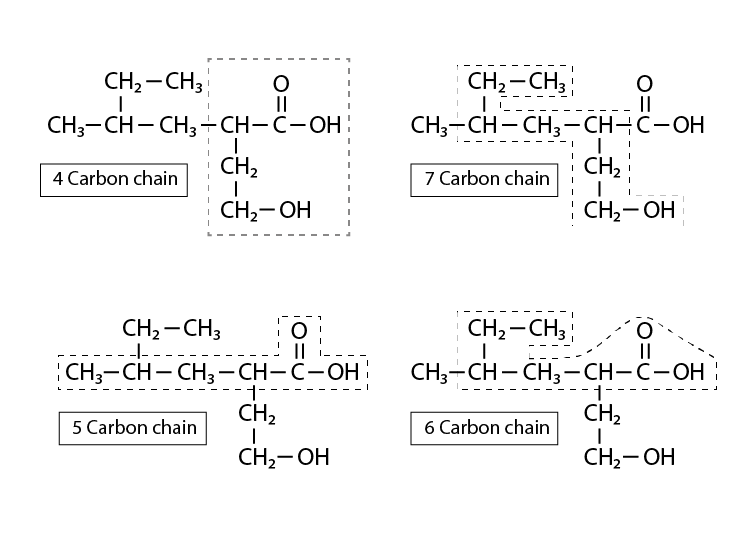a. The main(parent) chain should be the longest possible carbon chain with all the carbons with the functional groups included in them. The above selection is the only possible one with both the functional groups in the main chain.

b. Out of carboxylic acid and alcohol acid gets the priority hence the numbering starts from carboxylic carbon.

c. There is a branched alkyl group substitution at second carbon

d. There is a hydroxyl group at 4th carbon therefore it can be named as 2-(2-methyl butyl)-4-hydroxybutanoic acid.

33. In DNA and RNA, the nitrogen atom is present in the ring system. Can the Kjeldahl method be used for the estimation of nitrogen present in these? Give reasons.

Solution:

The Kjeldahl method cannot be used for the estimation of nitrogen present in DNA and RNA because nitrogen cannot be converted into ammonium sulphate in the conditions provided in this method. The difficulty in decomposing ring structures limits this test for DNA and RNA.

34. If a liquid compound decomposes at its boiling point, which method(s) can you choose for its purification. It is known that the compound is stable at low pressure, steam volatile and insoluble in water.

Solution:

As the liquid compound decomposes at its boiling point which means that the compound is heat-sensitive, hence we use “Steam distillation” for its purification. It is done for temperature-sensitive material.

Note: Answer the questions 35 to 38 based on the information given below:

“Stability of carbocations depends upon the electron releasing inductive effect

of groups adjacent to positively charged carbon atom involvement of

neighbouring groups in hyperconjugation and resonance.”

35. Draw the possible resonance structures forand predict which

of the structures is more stable. Give the reason for your answer.

Solution: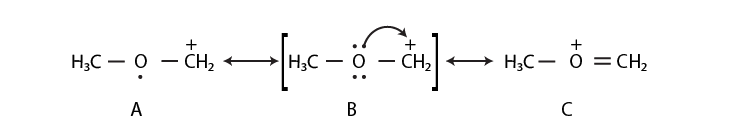The structure C is more stable than that of the structure A because, in structure C, the octet of all the atoms is complete, whereas, in structure A, C-atom having a positive charge is not having 8 electrons in its valence shell.

36. Which of the following ions is more stable? Use resonance to explain your answer.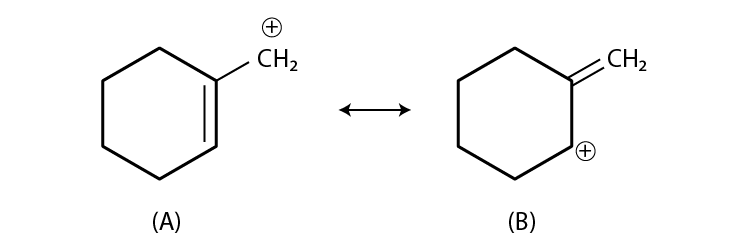Solution: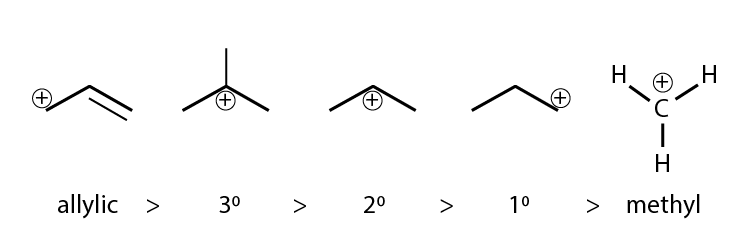The stability of carbocation is decided by inductive effect, hyperconjugation, resonance etc.

As structure A is having a carbocation, which is primary and allylic carbocation, whereas the carbocation in structure B has secondary and allylic carbocation.

Hence, structure B is more stable.

37. The structure of triphenylmethylcation is given below. This is very stable and some of its salts can be stored for months. Explain the cause of the high stability of this cation.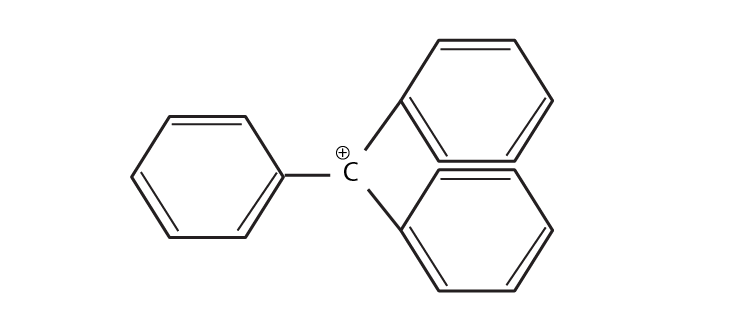Solution:

Triphenylcarbocation is tertiary carbocation and the positive charge is on the carbon atom which is stabilized by the three phenyl group by resonance. Due to the resonance the stability increases.

38. Write structures of various carbocation that can be obtained from 2-methyl butane. Arrange these carbocations in order of increasing stability.

Solution: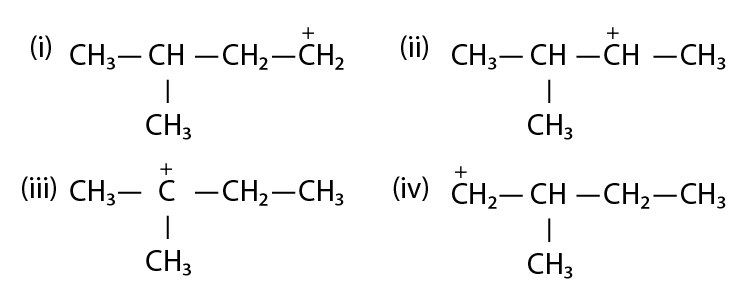the stability order is found to be:

(III) > (II) > (I) > (IV)

This is because (III) is tertiary carbocation, (II) is secondary carbocation, (I) and (IV) are primary,

39. Three students, Manish, Ramesh and Rajni were determining the extra

elements present in an organic compound given by their teacher. They

prepared the Lassaigne’s extract (L.E.) independently by the fusion of the

compound with sodium metal. Then they added solid FeSO4 and dilute

sulphuric acid to a part of Lassaigne’s extract. Manish and Rajni obtained

Prussian blue colour but Ramesh got a red colour. Ramesh repeated the test

with the same Lassaigne’s extract but again got red colour only. They were

surprised and went to their teacher and told him about their observation.

A teacher asked them to think over the reason for this. Can you help them by

giving the reason for this observation? Also, write the chemical equations to

explain the formation of compounds of different colours

Solution:

3NaCNS + FeSO4 + dilute sulphuric→ Fe(CNS)3 (Blood red colour) + 3Na+

Ramesh has Nitrogen as well as Sulphur present in his organic compound so, he was observed the Blood-red colour of Fe(CNS)3 and Manish and Rajni were having only Nitrogen in their compound, hence they got Prussian blue colour.

40. Name the compounds whose line formulae are given below: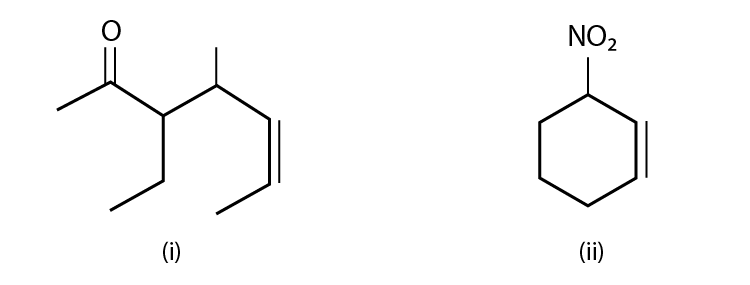Solution:

(i) The name of the compound is 3-ethyl-4-methyl-5-heptane-2-one.

(ii) The name of the compound is; 1-nitro-cyclohexane-2-ene.

41. Write structural formulae for compounds named as-

(a) 1-Bromoheptane

(b) 5-Bromoheptanoic acid

Solution:

(a)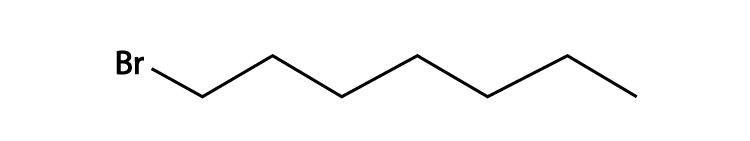(b)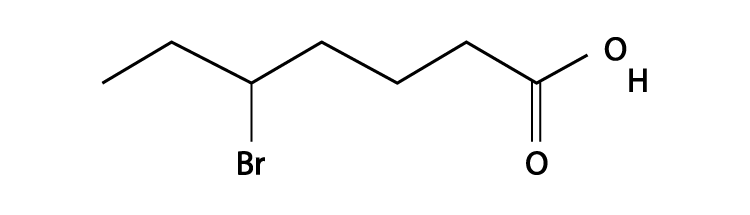42. Draw the resonance structures of the following compounds;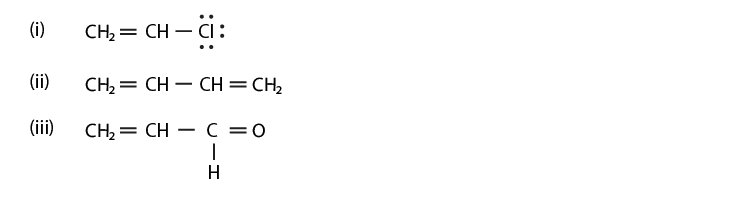Solution: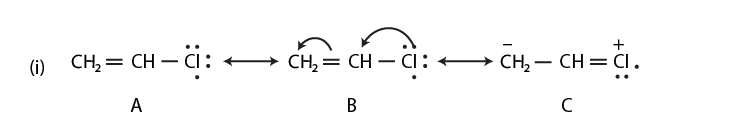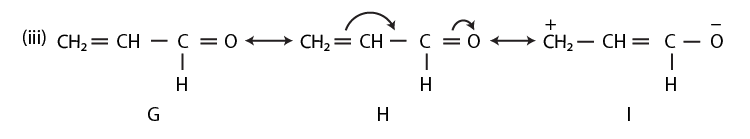43. Identify the most stable species in the following set of ions giving reasons: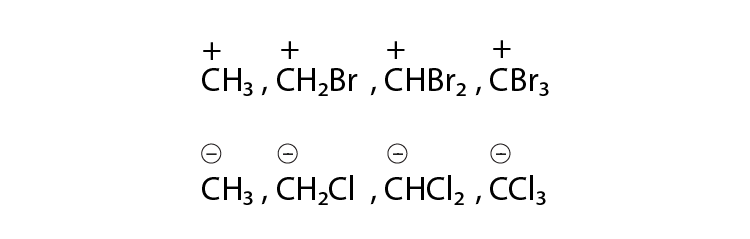Solution:

(i) C+H3 will be more stable as the bromine atom destabilizes the positive charge on a carbon atom. Bromine atom is an electron-withdrawing group and has a lone pair of electrons.

(ii) CCl3 will be most stable because chlorine is more electron-withdrawing atom and the negative charge on carbon will be stabilized by the chlorine atom. As the number of chlorine atom attached to carbocation increases the stability also increases.

44. Give three points of differences between the inductive effect and resonance effect.

Solution:

1. In the inductive effect, the transmission of the electron is only from sigma bond whereas in resonance effect the transmission occurs from both sigma and pi bond.

2. It is possible when the polarization of bond takes place whereas the resonance effect is possible only if the system is conjugated.

3. Electronegativity plays role in inductive effect whereas in resonance effect more number of an alternative double bond will lead to more resonating structure and hence more stability.

45. Which of the following compounds will not exist as a resonance hybrid. Give the reason for your answer:

Solution:

Conjugation is only possible when an atom has any of the charge or alternative position of the double bond. Here CH3OH will not exist as resonance hybrid because of the reason.

46. Why does SO3 act as an electrophile?

Solution:

Oxygen is more electronegative than sulphur and the electron density at S in SO3 is reduced and they need more electrons to suppress the partial positive charge and hence they act as an electrophile.

47. Resonance structures of propenal are given below. Which of these resonating structures is more stable? Give a reason for your answer.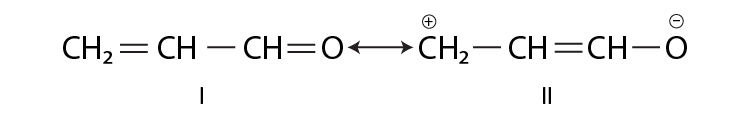Solution:

The structure I will be more stable than structure II because all the atoms are having complete octet in structure I and the carbon atom having positive charge don’t have a complete octet in structure II.

48. By mistake, an alcohol (boiling point 97°C) was mixed with a hydrocarbon (boiling point 68°C). Suggest a suitable method to separate the two compounds. Explain the reason for your choice.

Solution:

We can separate the mixture by steam distillation. Both alcohol and hydrocarbon don’t have much difference in the boiling point. Steam distillation is particularly used for the purification of temperature-sensitive material.

49. Which of the two structures (A) and (B) given below is more stabilised by resonance? Explain.

(A) CH3COOH (B) CH3COO-

Solution:

The two resonating structure in compound A, are not equivalent, whereas compound B, having a negative charge on the oxygen atom is having the two equivalent structures, hence the compound B is more stable.

IV. Matching Type

In the following questions, more than one correlation is possible between

options of Column I and Column II. Make as many correlations as you can.

50. Match the type of mixture of compounds in Column I with the technique of

separation/purification given in Column II.

 Column I (i) Two solids which have different solubilities in a solvent and which do not undergo a reaction when dissolved in it. (ii) The liquid that decomposes at its boiling point (iii) Steam volatile liquid (iv) Two liquids which have boiling points close to each other (v) Two liquids with the large difference in boiling points. Column II (a) Steam distillation (b) Fractional distillation (c) Simple distillation (d) Distillation under reduced pressure (e) Crystallisation

Solution:

(i) is e

(ii) is d

(iii) is a

(iv) is b

(v) is c

51. Match the terms mentioned in Column I with the terms in Column II.

 Column I (i) Carbocation (ii) Nucleophile (iii) Hyperconjugation (iv) Isomers (v) sp hybridisation (vi) Electrophile Column II (a) Cyclohexane and 1- hexene (b) Conjugation of electrons of C–Hσ bond with empty p-orbital present at adjacent positively charged carbon. (c) sp2 hybridised carbon with empty p-orbital (d) Ethyne (e) Species that can receive a pair of electrons (f) Species that can supply a pair of electrons.

Solution:

(i) is c

(ii) is f

(iii) is b

(iv) is a

(v) is d

(vi) is e

52. Match Column I with Column II.

 Column I (i) Dumas method (ii) Kjeldahl’s method (iii) Carius method (iv) Chromatography Column II (a) AgNO3 (b) Silica gel (c) Nitrogen gas (d) Free radicals (e) Ammonium sulphate

Solution:

(i) is c

(ii) is e

(iii) is a

(iv) is b

(v) is d

53. Match the intermediates given in Column I with their probable structure in

Column II.

 Column I (i) Free radical (ii) Carbocation (iii) Carbanion Column II (a) Trigonal planar (b) Pyramidal (c) Linear

Solution:

(i) is a

(ii) is a

(iii) is b

54. Match the ions given in Column I with their nature-given in Column II.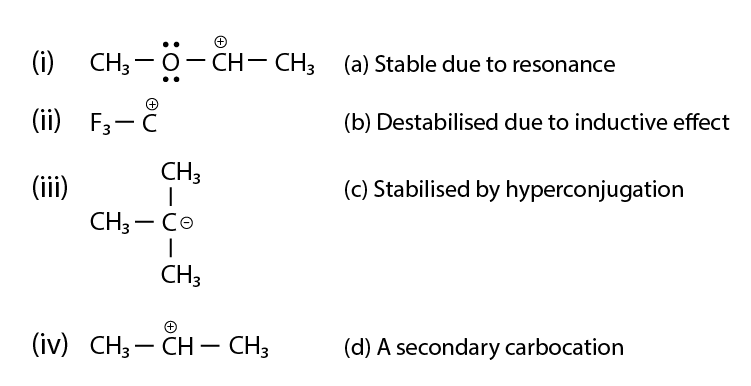Solution:

(i) is a, b and d

(ii) is b

(iii) is b

(iv) are c and d

#### Subtopics of Chapter 12 Organic Chemistry Some Basic Principles and Techniques

1. General Introduction
2. Tetravalence Of Carbon: Shapes Of Organic Compounds
3. Structural Representations Of Organic Compounds
1. Complete, Condensed And Bond-line Structural Formulas
2. Three-dimensional Representation Of Organic Molecules
4. Classification Of Organic Compounds
5. Nomenclature Of Organic Compounds
1. The Iupac System Of Nomenclature
2. Iupac Nomenclature Of Alkanes
3. Nomenclature Of Organic Compounds Having Functional Group(S)
4. Nomenclature Of Substituted Benzene Compounds
6. Isomerism
1. Structural Isomerism
2. Stereoisomerism
7. Fundamental Concepts In Organic Reaction Mechanism
1. Fission Of A Covalent Bond
2. Nucleophiles And Electrophiles
3. Electron Movement In Organic Reactions
4. Electron Displacement Effects In Covalent Bonds
5. Inductive Effect
6. Resonance Structure
7. Resonance Effect
8. Electromeric Effect (E Effect)
9. Hyperconjugation
10. Types Of Organic Reactions And Mechanisms
8. Methods Of Purification Of Organic Compounds
1. Sublimation
2. Crystallisation
3. Distillation
4. Differential Extraction
5. Chromatography
9. Qualitative Analysis Of Organic Compounds
1. Detection Of Carbon And Hydrogen
2. Detection Of Other Elements
10. Quantitative Analysis
1. Carbon And Hydrogen
2. Nitrogen
3. Halogens
4. Sulphur
5. Phosphorus
6. Oxygen.

To learn the concepts of Organic Chemistry students should solve NCERT Exemplar Class 11 Chemistry Chapter 12.

## Frequently Asked Questions on NCERT Exemplar Solutions for Class 11 Chemistry Chapter 12

### Which are the important topics I should learn from the Chapter 12 of NCERT Exemplar Solutions for Class 11 Chemistry?

The important topics you should learn from the Chapter 12 of NCERT Exemplar Solutions for Class 11 Chemistry are –
General Introduction
Tetravalence Of Carbon: Shapes Of Organic Compounds
Structural Representations Of Organic Compounds
Complete, Condensed And Bond-line Structural Formulas
Three-dimensional Representation Of Organic Molecules
Classification Of Organic Compounds
Nomenclature Of Organic Compounds
The Iupac System Of Nomenclature
Iupac Nomenclature Of Alkanes
Nomenclature Of Organic Compounds Having Functional Group(S)
Nomenclature Of Substituted Benzene Compounds
Isomerism
Structural Isomerism
Stereoisomerism
Fundamental Concepts In Organic Reaction Mechanism
Fission Of A Covalent Bond
Nucleophiles And Electrophiles
Electron Movement In Organic Reactions
Electron Displacement Effects In Covalent Bonds
Inductive Effect
Resonance Structure
Resonance Effect
Electromeric Effect (E Effect)
Hyperconjugation
Types Of Organic Reactions And Mechanisms

### Give three points of differences between the inductive effect and resonance effect in the Chapter 12 of NCERT Exemplar Solutions for Class 11 Chemistry?

1. In the inductive effect, the transmission of the electron is only from the sigma bond whereas in the resonance effect the transmission occurs from both sigma and pi bonds.
2. It is possible when the polarization of bond takes place whereas the resonance effect is possible only if the system is conjugated.
3. Electronegativity plays a role in inductive effect whereas in resonance effect more number of an alternative double bond will lead to more resonating structure and hence more stability.

### Why should I download the NCERT Exemplar Solutions for Class 11 Chemistry Chapter 12 from BYJU’S?

Students who aspire to score good marks in the board exams and other competitive exams should refer to the NCERT Solutions from BYJU’S. It can be considered as a solutions book which helps students in getting their doubts cleared instantly. Students will be able to obtain a comprehensive knowledge from the solutions PDF available at BYJU’S. While answering the textbook questions, students can cross check their answer with the solutions and understand the areas which they are lagging behind.

For NCERT notes, study material, previous year question papers, sample papers, videos, animations, NCERT solutions, NCERT exemplar’s, books, worksheets and exercises for all the classes and subjects, visit BYJU’S website or download BYJU’S learning App for an effective learning experience.